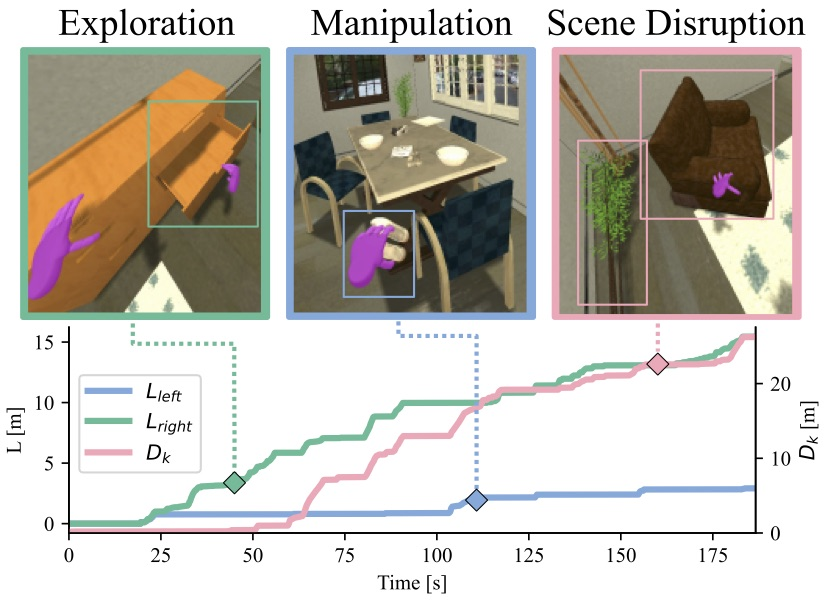# Metrics

We used a combination of metrics to measure the performance of the agent, a primary metric based on the degree of completion of the activity, and a set of secondary metrics that evaluate different aspects of the efficiency of the solution.

## Primary Metric: Degree of Success

The primary metric for BEHAVIOR is the (maximum) fraction of satisfied logical predicates of the activity’s goal definition (Q). In the case that a goal is multi-part (e.g., “put one apple on a table or put two pears on a table”), Q is automatically calculated by choosing the combination of goal conditions that maximize Q.## Secondary Metrics: Efficiency

The secondary metrics capture how efficiently an agent performs the activity relative to the best human demonstration.

• Simulated time (Tsim): The total simulated (not wall-clock) time, how quickly the agent solves the activity.

• Kinematic disarrangement (DK): Displacement caused by the agent in the environment. This can be accumulated over time, or differential i.e. computed between two timesteps, e.g. initial, final.

• Logical disarrangement, DL: Amount of changes caused by the agent in the logical state of the environment. This can be accumulated over time or differential between two time steps. This captures kinematic state changes (putting an item on top of another item, taking an item out of a cupboard) as well as non-kinematic state changes like toggling a stove on, making an item wet, freezing an item. All kinematic state changes are grouped as 1 unit of logical disarrangement per item.

• Distance navigated

• Lbody: Accumulated distance traveled by the agent’s base body. This metric evaluates the efficiency of the agent in navigating the environment.

• Displacement of hands, Lleft and Lright: Accumulated displacement of each of the agent’s hands while in contact with another object for manipulation (i.e., grasping, pushing, etc). This metric evaluates the efficiency of the agent in its interaction with the environment.If you want to compute the metrics on your own, it is recommended that you follow the example in `igibson/examples/behavior/behavior_env_metrics.py`. This example shows how to leverage the start/step/end callbacks and aggregator to collect metrics on a behavior run.

Secondary metrics can be computed in three different modes:

• per `timestep` – this provides the raw value of a metric for a given timestep

• `integrated` over time – this is the sum of the absolute value of the metric over time

• `relative` change between two timesteps – this is the difference of the per timestep metric between the start and the current timestep

The following is a description of the report of metrics returned at the end of an episode. For additional information, see the corresponding implementation under `iGibson/metrics`.

• `q_score` – Primary metric: Degree of Success

• `timestep`

• `integrated`

• `kinematic_disarrangement` – see above

• `timestep`

• `integrated`

• `relative`

• `logical_disarrangment` – see above

• `relative`

• `total_objects`

• `total_states`

• `agent_distance` – distance traveled by torso of the behavior_robot

• `timestep`

• `integrated`

• `grasp_distance` – distance traveled by manipulators of behavior_robot while grasping

• `left_hand`/`right_hand`/`body`

• `timestep`

• `integrated`

• `work` – F*d per frame performed by all body parts

• `left_hand`/`right_hand`/`body`

• `timestep`

• `integrated`

• `pos` – 3D position of agent parts

• `left_hand`/`right_hand`/`body`

• `timestep`

• `integrated`

• `local_pos` – 3D position of agent parts in frame of reference of body

• `left_hand`/`right_hand`

• `timestep`

• `integrated`

• `grasping` – Whether hand is grasping (or touching) object

• `left_hand`/`right_hand`

• `timestep`

• `integrated`

• `reset` – Demos allow a user to reset the bullet position of a robot part to the VR controller position to avoid getting “stuck” on objects

• `left_hand`/`right_hand`/`body`

• `timestep`

• `integrated`

• `satisfied_predicates` – the top-level satisfied goal indices in the activity goal condition

• `timestep`

• `integrated`

• `time` – simulator time in both simulation steps and simulated time

• `simulator_steps`

• `simulator_time`

Note that computing some of these metrics will strongly affect your runtime performance as they query simulator state, so it is recommended to only compute them in your evaluation, not during traning.